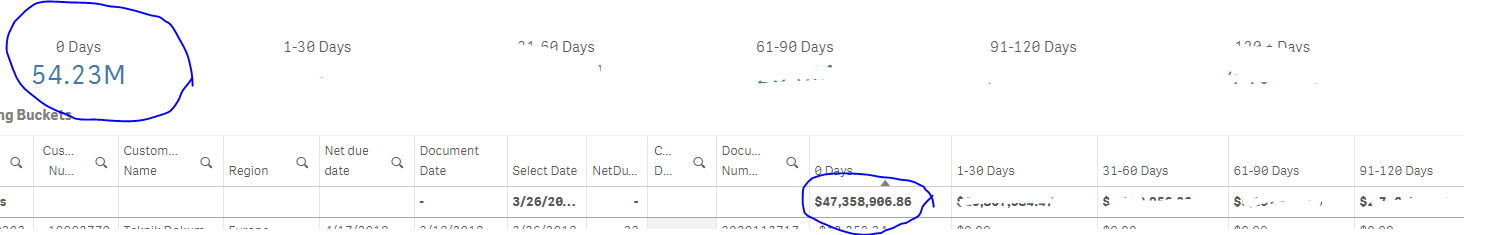# New to Qlik Sense

Discussion board where members can get started with Qlik Sense.

Announcements
Save \$300 - Register for QlikWorld (formerly Qonnections) by January 31st: Learn More
Highlighted
New Contributor III

## KPI is not same as totals in tableThe expression I used to calculate 0 days is

=sum(if (\$(vClearing) and num(\$(vCalendarDate)) - num([Net due date]) <= 0  , [Debit/Credit Amount],0))

when I use the same expression in KPI it gives me a different total.

any help would be appreciated!

3 Replies
New Contributor III

## Re: KPI is not same as totals in table

=sum(if (\$(vClearing) and num(\$(vCalendarDate)) - num([Net due date]) <= 0 ....

this is correty and?

maybe this....

=sum(if (num(\$(vClearing)) = (num(\$(vCalendarDate)) - num([Net due date])) <= 0 .....Partner

## Re: KPI is not same as totals in table

Sample qvf would help.

ThanksMVP

## Re: KPI is not same as totals in table

can you post variable definition?

Just guess I think you required aggr()

Sum(aggr(sum(if (\$(vClearing) and num(\$(vCalendarDate)) - num([Net due date]) <= 0  , [Debit/Credit Amount],0)),YourAllDimensionIncludedInChart)).

Regards,

Great dreamer's dreams never fulfilled, they are always transcended.